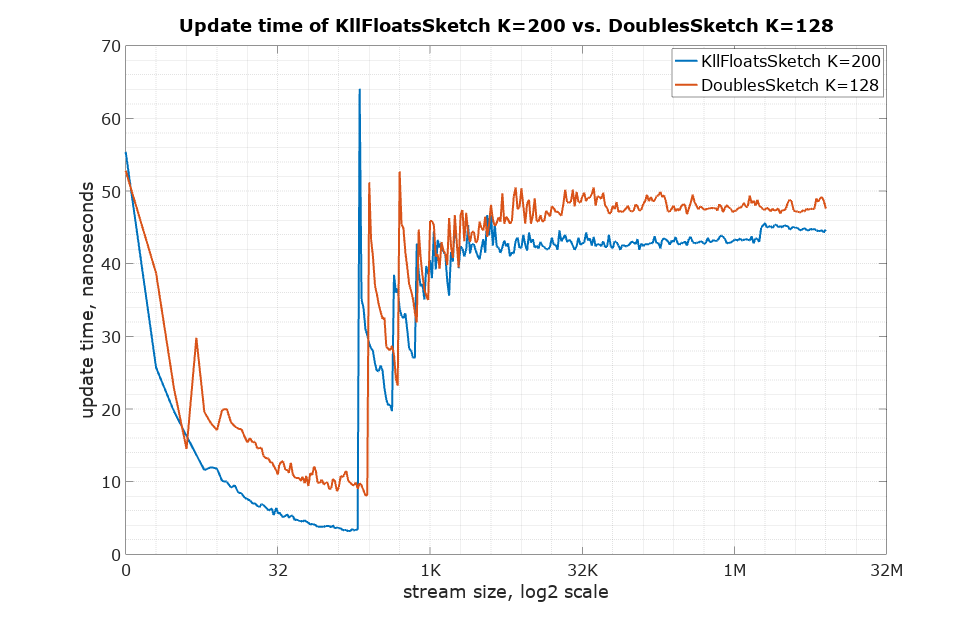## KLL Sketch

Implementation of a very compact quantiles sketch with lazy compaction scheme and nearly optimal accuracy per bit. See Optimal Quantile Approximation in Streams, by Zohar Karnin, Kevin Lang, Edo Liberty. The name KLL is composed of the initial letters of the last names of the authors.

The usage of KllSketch is very similar to the classic quantiles DoublesSketch.

• The key feature of this sketch is its compactness for a given accuracy.
• It is separately implemented for both float and double values and can be configured for use on-heap or off-heap (Direct mode).
• The parameter K that affects the accuracy and the size of the sketch is not restricted to powers of 2.
• The default of 200 was chosen to yield approximately the same normalized rank error (1.65%) as the classic quantiles DoublesSketch (K=128, error 1.73%).

### Java example

import org.apache.datasketches.kll.KllFloatsSketch;

KllFloatsSketch sketch = KllFloatsSketch.newHeapInstance();
int n = 1000000;
for (int i = 0; i < n; i++) {
sketch.update(i);
}
float median = sketch.getQuantile(0.5);
double rankOf1000 = sketch.getRank(1000);


### Differences of KllSketch from the original quantiles DoublesSketch

• KLL has a smaller size for the same accuracy.
• KLL is slightly faster to update.
• The KLL parameter K doesn’t have to be power of 2.
• KLL operates with either float values or double values.
• KLL uses a merge method rather than a union object.

The starting point for the comparison is setting K in such a way that rank error would be approximately the same. As pointed out above, the default K for both sketches should achieve this. Here is the comparison of the single-sided normalized rank error (getRank() method) for the default K: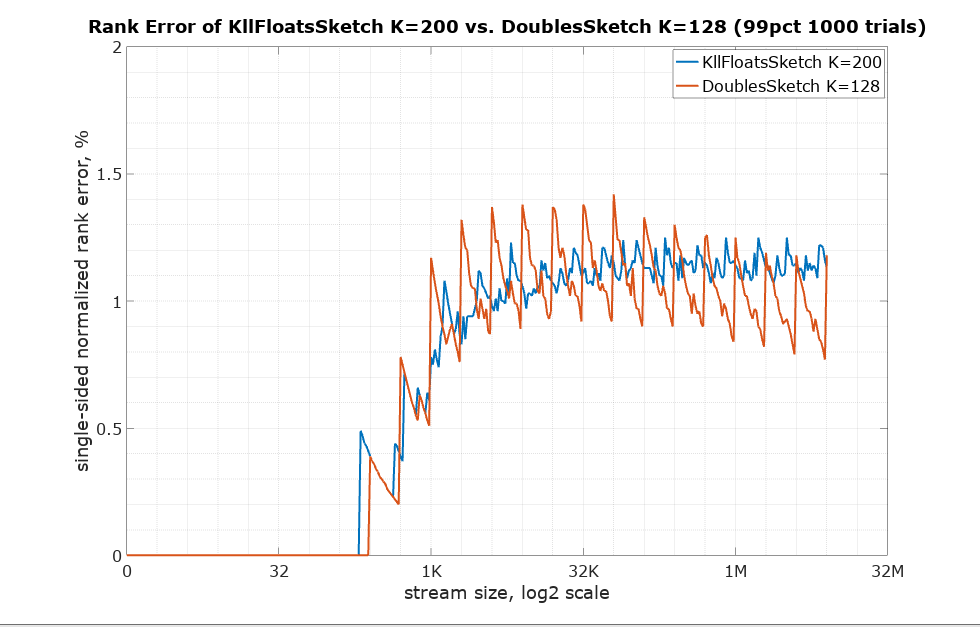The classic quantiles DoublesSketch has two forms with different serialized sizes: UpdateDoublesSketch and CompactDoublesSketch. The KLL sketches makes this distinction differently. When the KllSketch is serialized using toByteArray() it is always in a compact form and immutable. When the KllSketch is on-heap it is always updatable. It can be created off-heap using the static factory method newDirectInstance(…) method, which is also updatable. It is possible to move from off-heap (Direct) to on-heap using the heapify(Memory) method. The merge(…) method will work with off-heap sketches, on-heap sketches and Memory wrapped compact byte arrays.

Here is the comparison of serialized sizes: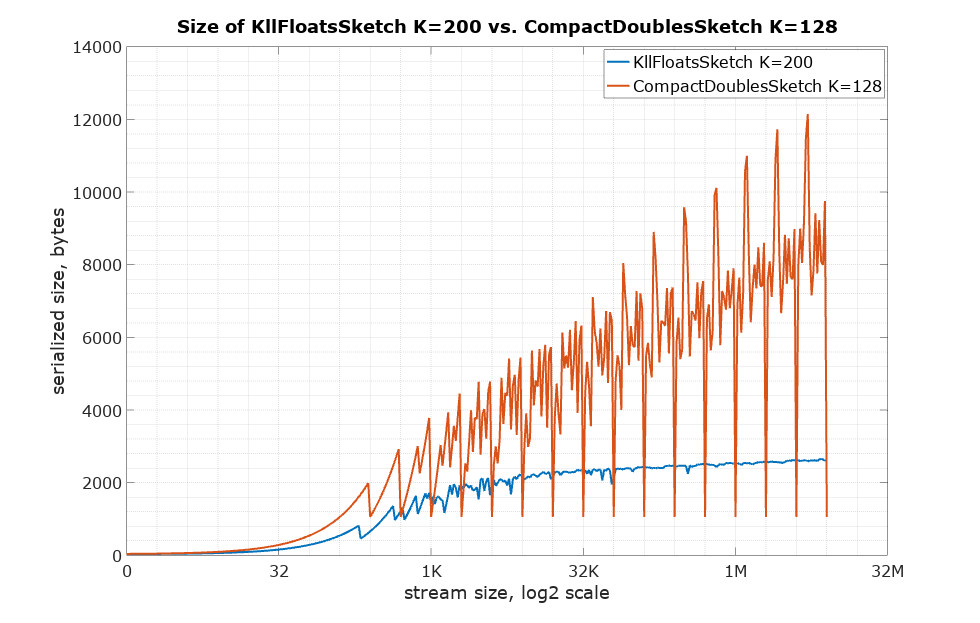Here is the comparison of the number of retained items to see the difference with no influence of the size of the item type: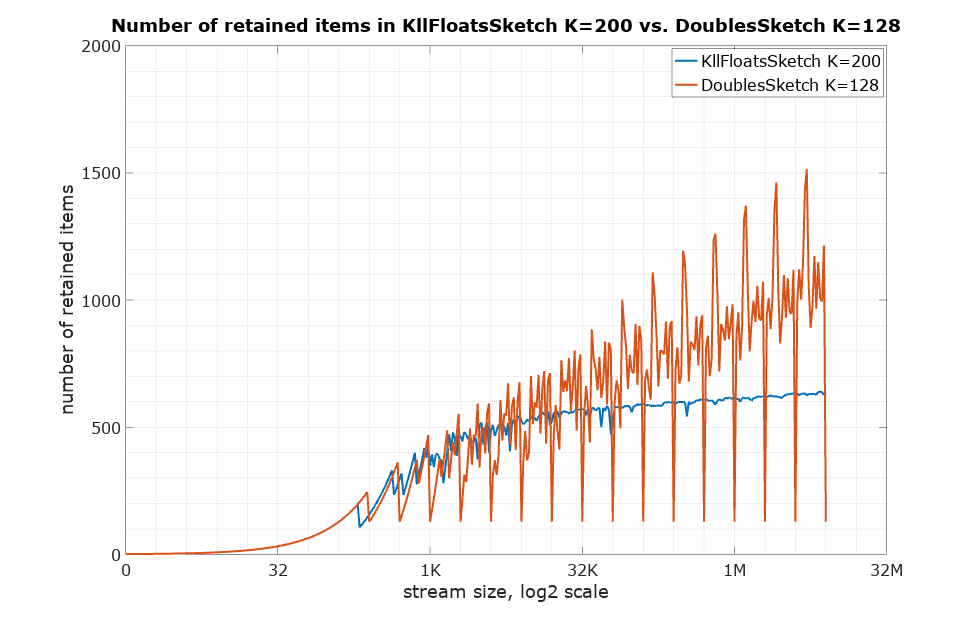Below is the accuracy per byte measure (the higher the better). Suppose rank error is 1%, so the corresponding accuracy would be 99%. Divide that by size in bytes to arrive at this measure of how expensive each percentage point is in terms of space needed: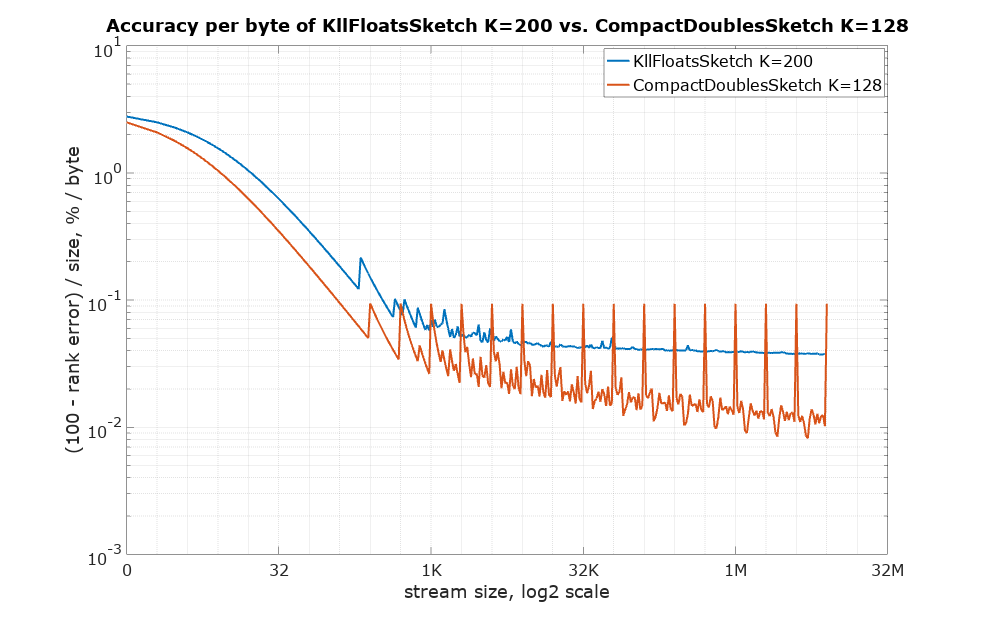Below is the update() method speed: